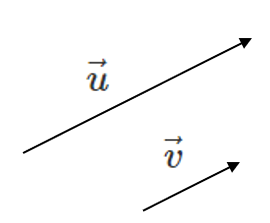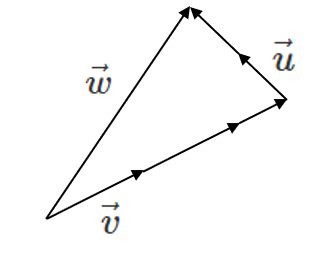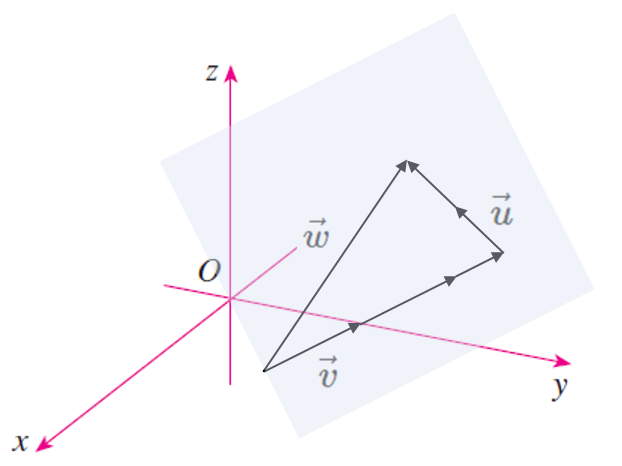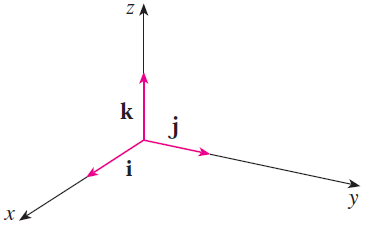# Linear Combinations

A vector is said to be a Linear Combination of another vector if there exists a non-zero scalar such that $$\vec{u} = k\vec{v}$$ or $$\vec{u} = a\vec{v} + b\vec{w}$$ etc. In general:

$$\vec{u} = \sum_{i=0}^n a_i\vec{v}_i = a_1\vec{v}_1 + a_2\vec{v}_2 + \cdots + a_n\vec{v}_n$$

Where $$a_1, a_2,$$ etc. are scalars and $$\vec{v}_1, \vec{v}_2,$$ etc. are vectors.

If $$\vec{u} = k\vec{v}$$, $$\vec{u}$$ is said to be Collinear with respect to $$\vec{v}$$. In other words, $$\vec{u}$$ is a scalar multiple of $$\vec{v}$$, $$\vec{u}$$ is parallel to $$\vec{v}$$ and $$\vec{u}$$ and $$\vec{v}$$ point in the same direction.$$\vec{u} \parallel \vec{v}$$

$$\vec{u} = 2.5 \vec{v}$$

If $$\vec{u} = a\vec{v} + b\vec{w}$$, $$\vec{u}$$ is said to be Coplanar with respect to $$\vec{v}$$ and $$\vec{w}$$. In other words, $$\vec{u}$$, $$\vec{v}$$ and $$\vec{w}$$ all exist on the same plane in 3-D space.$$\vec{w} = 2.5 \vec{v} + 2 \vec{u}$$

In 3-D, imagine that all three vectors can be drawn on a piece of paper.Are the following vectors collinear? $$\vec{u} = \left< 1,4,5 \right>,\vec{v} = \left< -2,-8,10 \right>$$

What is the value of $$z$$ to make the vectors collinear? $$\vec{u} = \left< 1,4,5 \right>,\vec{v} = \left< -2,-8,z \right>$$

## Standard Basis Form

Recall the Standard Basis unit vectors that are in the direction of the the x, y and z axes:

$$\hat{\imath} = \left<1,0,0\right>$$

$$\hat{\jmath} = \left<0,1,0\right>$$

$$\hat{k} = \left<0,0,1\right>$$We can express any vector in 3-D as a linear combination of these vectors. This is called Standard Basis Form

Express the vector $$\vec{u} = \left< 120, -45, 5000 \right>$$ as a linear combination of the Standard Basis unit vectors $$\hat{\imath}, \hat{\jmath}$$ and $$\hat{k}$$.

Can $$\vec{u} = \left<2,4,6 \right>$$ be written as a linear combination of $$\vec{a} = \left<1,6 \right>$$ and $$\vec{b} = \left<-5,16 \right>$$?